# Research Methods For Public Health Assignment Sample

6500 Project Delivered
4.8/5 User Rating

## Research Methods For Public Health Assignment Sample

• Type Assignment
• Pages235

## Introduction

Get free samples written by our Top-Notch subject experts for taking online Assignment Help services.

### 1. Section A

#### 1.1. Present summary descriptive statistics for demographic variables (age, gender, weight, height) at baseline, for the group as a whole, and by diet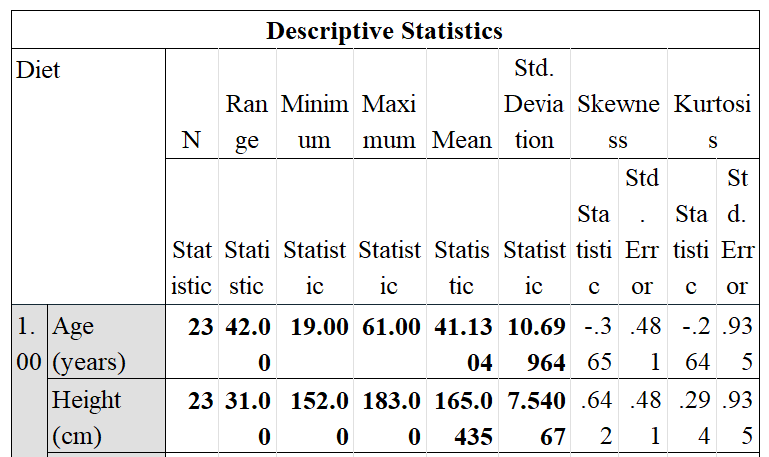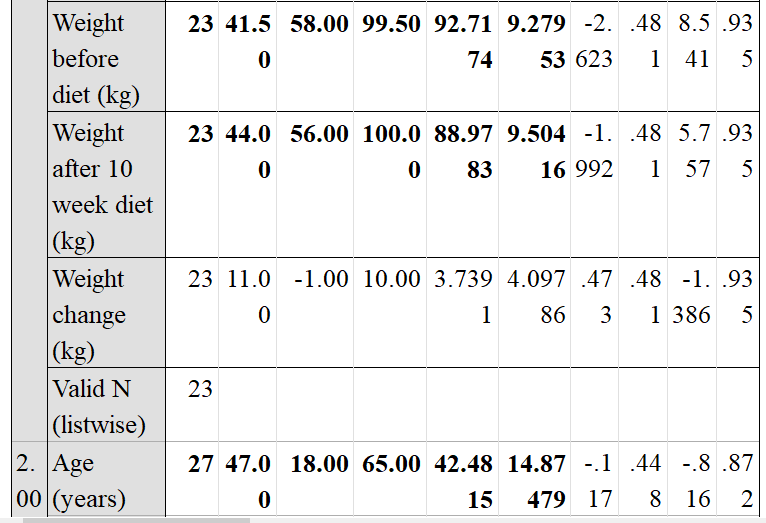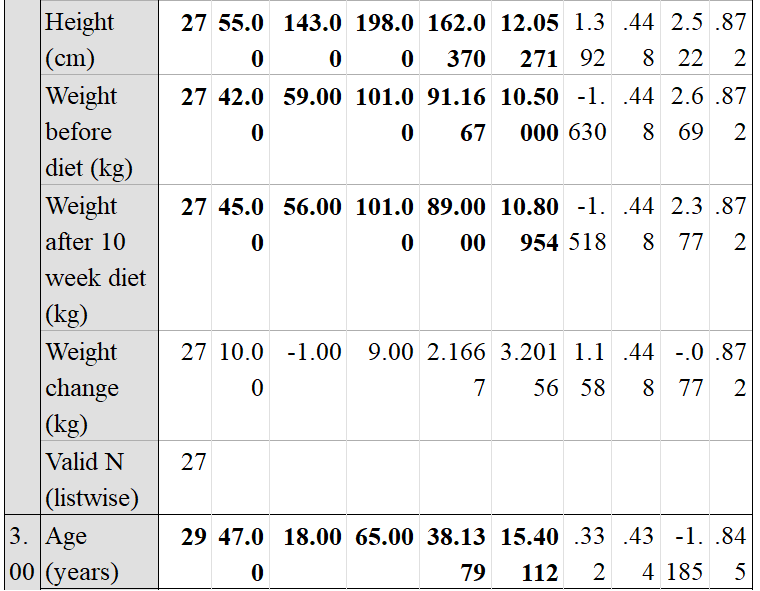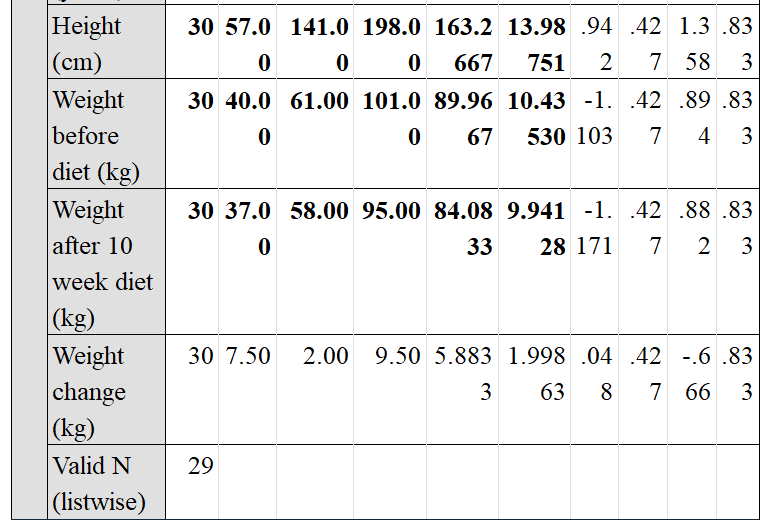This presented statistical table of sample statistics contains data on overall weight change, and table three contains information on the three different diet regimes and their relationship to people's demographic status. In this table of diet number 1.00, for the factor of age, the N statical value is 23, the range statistical value is 42.00, minimum statistic value is 19.00, and maximum statistic value is 61.00, where the mean statistic value is 41.1304, and the value of standard deviation is 10.69964. In the diet number 1.00, for the factor of height, the N statical value is 23, the range statistical value is 31.00, “minimum statistic value” is 152.00, and highest statistic value is 183.00, where the mean statistic value is 165.0435, and the value of standard deviation is 7.54067. In the diet number 1.00, for the factor of weight, the N statical value is 23, the range statistical value is 41.50, “minimum statistic value” is 58.00, and “maximum statistic value” is 99.50, where the mean statistic value is 92.7174, and the value of standard deviation is 9.27953 (Little and Rubin, 2019). In the diet number 1.00, for the factor of weight after 10 week of diet, the N statical value is 23, the range statistical value is 44.00, “minimum statistic value” is 56.00, and maximum statistic value is 100.00, where the mean statistic value is 88.9783, and the value of standard deviation is 9.50416.

In this table of diet number 2.00, for the factor of age, the N statical value is 27, the range statistical value is 47.00, “minimum statistic value” is 18.00, and maximum statistic value is 65.00, where the mean statistic value is 42.4815, and the value of standard deviation is 14.87479. In the diet number 1.00, for the factor of height, the N statical value is 27, the range statistical value is 55.00, “minimum statistic value” is 143.00, and “maximum statistic value” is 198.00, where the mean statistic value is 162.0370, and the value of standard deviation is 12.05271. In the diet number 1.00, for the factor of weight, the N statical value is 27, the range statistical value is 42.00, least statistic value is 59.00, and maximum statistic value is 101.00, where the mean statistic value is 91.1667, and the value of standard deviation is 10.50000 (Fagerlandet al. 2017). In the diet number 1.00, for the factor of weight after 10 week of diet, the N statical value is 27, the range statistical value is 45.00, “minimum statistic value” is 56.00, and maximum statistic value is 101.00, where the mean statistic value is 8.0000, and the value of standard deviation is 10.80954.

In this table of diet number 3.00, for the factor of age, the N statically value is 29, the range statistical value is 47.00, “minimum statistic value” is 18.00, and “maximum statistic value” is 65.00, where the mean statistic value is 38.1379 and the value of standard deviation is 15.40112. In the diet number 1.00, for the factor of height, the N statical value is 30, the range statistical value is 57.00, “minimum statistic value” is 141.00, and “maximum statistic value” is 198.00, where the mean statistic value is 163.2667, and the value of standard deviation is13.98751. In the diet number 1.00, for the factor of weight, the N statical value is 30, the range statistical value is 40.00, minimum statistic value is 61.00, and “maximum statistic value” is 101.00, where the mean statistic value is 89.9667, and the value of standard deviation is 10.43530 (Schabenberger and Gotway, 2017). In the diet number 1.00, for the factor of weight after 10 week of diet, the N statical value is 30, the range statistical value is 37.00, minimum statistic value is 58.00, and maximum statistic value is 95.00, where the mean statistic value is 84.0833, and the value of standard deviation is 9.94128.

#### 1.2. What are the findings from the statistical test (pre vs post) to see if the weight has been lost across the group as a whole?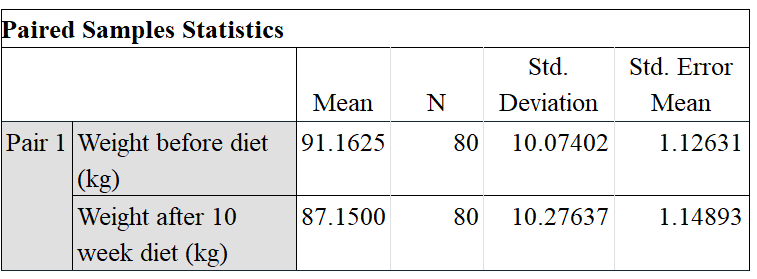There is data about weight before diet and weight after diet control in this presented statistical table of sample statistics. This statistical table shows the weight results for two alternative scenarios, namely weight before diet management and weight after diet control. Here in this table, the weight has been calculated in kg, as per the others data. In this paired sample statistics, for the factor of weight before the diet, the mean value is 91.1625 and the N value is 80, where the standard deviation for this is 10.07402, and the mean value of standard deviation is 1.12631 (Goss-Sampson, 2019). In this paired sample statistics, for the factor of weight after a diet, the mean value is 87.1500 and the N value is 80, where the standard deviation for this is 10.27637, and the mean value of standard deviation is 1.14893.

#### 1.3. What are the findings from the statistical test to determine which of the 3 diets was best for Losing weight?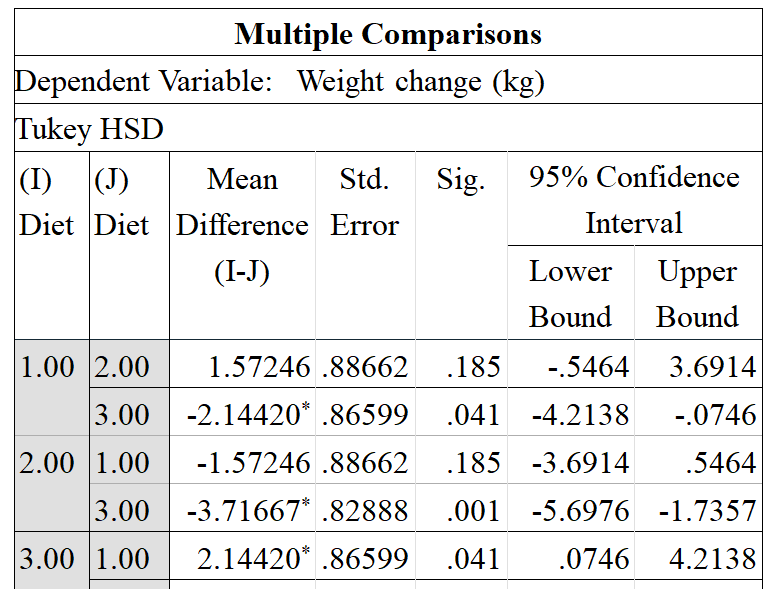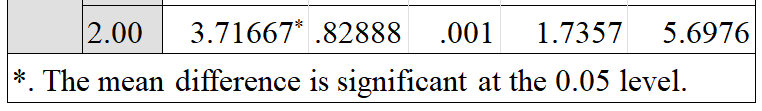The data on weight change in three different weight change diets is shown in this statistical table of sample statistics, with the major focus on the three different diet strategies and their effects on people. In the comparison table of diet method for the J diet of 2.00 under the I diet of 1.00, the mean difference is 1.57246 and the standard error is .88662 where the sig value is .185, lower bound value is -.5464 and the upper bound value is 3.6914. For the J diet of 3.00 under the I diet of 1.00, the mean difference is -2.14420 and the standard error is .86599 where the sig value is .041, the lower bound value is -4.2138 and the upper bound value is -.0746. In the comparison table of diet method for the J diet of 2.00 under the I diet of 1.00, the mean difference is 1.57246 and the standard error is .88662 where the sig value is .185, lower bound value is -3.6914 and the upper bound value is .5464 (Gallardo?Lacourt, et al. 2018). For the J diet of 3.00 under the I diet of 2.00, the mean difference is -3.71667 and the standard error is .82888 where the sig value is .001, the lower bound value is -5.6976 and the upper bound value is -1.7357. In the comparison table of diet method for the J diet of 1.00 under the I diet of 3.00, the mean difference is 2.14420 and the standard error is .86599 where the sig value is .041, lower bound value is -0746 and the upper bound value is 42138. For the J diet of 2.00 under the I diet of 3.00, the mean difference is 3.71667 and the standard error is .82888 where the sig value is .001, the lower bound value is 1.7357 and the upper bound value is 5.6976.

#### 1.4. What are the findings from the statistical test to see whether there are gender differences for Weight loss?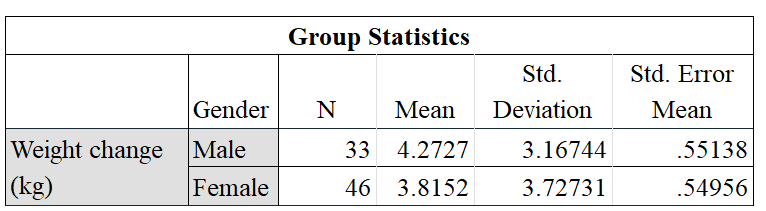There is data about weight change in this presented statistical table of sample statistics, and the weight measurement is done in the form of kg. In this presented statistical table of the weight change for the malefactor, the N value is 33, and the mean value is 4.2727, where the value of standard deviation is 3.16744 and the mean value of standard deviation is .55138 (Waroquiers, et al. 2017). In this presented statistical table of the weight change for the female factor, the N value is 46, and the mean value is 3.8152, where the value of standard deviation is 3.72731 and the mean value of standard deviation is .54956.

### 2. Section B

According to this presented statistical table of sample statistics, there is data regarding the weight change as a whole, and in this table three is a detail about the three different diet methods and their relation with the demographic status of the people. This table implies that weight has been significantly reduced after the diet control, where diet number 3 is the best diet compared to diet number 1 and diet number 2. According to this presented statistical table of sample statistics, there is data regarding the weight before diet and weight after the diet control (Xia, et al. 2017). This statistical table contains the result of the weight for the two different situations, where the two different situations are weight before the diet control and weight after the diet control. This table implies that weight has been significantly reduced after the diet control.

According to this presented statistical table of sample statistics, there is data regarding the weight change in three different weight change diets, where the main focus will be on the three different diet methods and their effects on the people. This table implies that diet number 3 is the best diet compared to diet number 1 and diet number 2 because the standard error of diet number 3 is less than diet number 1 and diet number 2. According to this presented statistical table of sample statistics, there is data regarding the weight change and the weight measurement is taken place in the form of kg (Härdle and Simar, 2019). This table implies that the rate of females is higher than the rate of males, where the weight change is more important for the women compared to the male because diet has had more impact on the female.

#### 2.1. Understanding of probability and statistical significance

Statistical significance mainly refers to the determination that is made by the analyst that implies that the specific results in the specific data are explained by the chance and hypothesis. Statistical hypothesis refers to the specific method of hypothesis testing through which the analyst makes the value of determination, where the test provides a p-value or the probability of the result observation based on the data. It is the assumption that results are truly for the chance alone, where a p-value of less than 5 percent or 5 percent is mainly considered as the statistical significance. It is mainly the determination of a relationship between the two or sometimes more than two variables that are caused by something other instead of chance. They are mainly used for providing the evidence of the null hypothesis where they hypothesize that there is no more than random chance in data work (Nordhaus and Moffat, 2017). The testing of the statistical hypothesis is mainly used for determining whether the result set is statistically significant or not.

#### 2.2. How to choose statistical tests and theory about the tests

Choosing the right statistical test is essential to test the right statistics for the research project, where it determines the effectiveness success of the statistical test in the research project. For a specific statistical test, the sample size needs to be sufficiently large for approximately or the true distribution of the specific population that has been studied for the research project (Stiglic, et al. 2019). There are two main criteria to choose the right statistical test for the test in a research project; they are highlighted below.
There is a need to see whether the data will meet a certain assumption or not, and through this basis, the statistical test needs to be chosen for the test.
There is a need to check the types of collected data that will be dealt with for the research project, and on that basis, the statistical test needs to be chosen for the test in the research project.

#### 2.3. Assumptions underlying parametric and non-parametric tests

Parametric tests mainly refer to those tests that are mainly based on the assumption regarding the population distribution and its parameters from which the sample size is mainly drawn. This is mainly often the assumption where the population is mainly distributed (Wasay, et al. 2017). And the Non-parametric tests are those texts that are free with any distribution of the population where they are mainly used for the variables of non-normal. Non-parametric tests are also valid for both the data normally distributed and also the data that are non-normally distributed.

#### 2.4. Levels of measurement

Levels of measurement refer to the informative and the interval measurement. There are mainly four types of measurement such as interval, nominal, ratio, and ordinal (Heradio, et al. 2019). These four different measurements are highlighted below in detail.
Nominal measurement refers to the data that can be categorized only.
Ordinal measurement refers to the data that can be categorized and along with that the data can be ranked.
Interval measurement refers to the data that can be categorized and ranked, along with that the data can be spaced also.
Ratio measurement refers to the data that can be categorized and ranked, along with that the data can be spaced also. Along with the space the data also has the neutral zero.

Reference list

Book
Fagerland, M., Lydersen, S. and Laake, P., 2017. Statistical analysis of contingency tables. CRC press.
Little, R.J. and Rubin, D.B., 2019. Statistical analysis with missing data (Vol. 793). John Wiley & Sons.
Schabenberger, O. and Gotway, C.A., 2017. Statistical methods for spatial data analysis. CRC press.

Journal
Gallardo?Lacourt, B., Nishimura, Y., Donovan, E., Gillies, D.M., Perry, G.W., Archer, W.E., Nava, O.A. and Spanswick, E.L., 2018. A statistical analysis of STEVE. Journal of Geophysical Research: Space Physics, 123(11), pp.9893-9905.
Goss-Sampson, M., 2019. Statistical analysis in JASP: A guide for students.
Härdle, W.K. and Simar, L., 2019. Applied multivariate statistical analysis (pp. 431-442). Springer International Publishing.
Heradio, R., Fernandez-Amoros, D., Mayr-Dorn, C. and Egyed, A., 2019, May. Supporting the statistical analysis of variability models. In 2019 IEEE/ACM 41st International Conference on Software Engineering (ICSE) (pp. 843-853). IEEE
Nordhaus, W.D. and Moffat, A., 2017. A survey of global impacts of climate change: replication, survey methods, and a statistical analysis.
Stiglic, G., Watson, R. and Cilar, L., 2019. R you ready? Using the R programme for statistical analysis and graphics. Research in nursing & health, 42(6), pp.494-499.
Waroquiers, D., Gonze, X., Rignanese, G.M., Welker-Nieuwoudt, C., Rosowski, F., Go?bel, M., Schenk, S., Degelmann, P., André, R., Glaum, R. and Hautier, G., 2017. Statistical analysis of coordination environments in oxides. Chemistry of Materials, 29(19), pp.8346-8360.
Wasay, A., Wei, X., Dayan, N. and Idreos, S., 2017, May. Data canopy: Accelerating exploratory statistical analysis. In Proceedings of the 2017 ACM International Conference on Management of Data (pp. 557-572).
Xia, Y., Sun, J. and Chen, D.G., 2018. Statistical analysis of microbiome data with R (Vol. 847). Singapore: Springer.

• Improved Scores

Get Better Grades In Every Subject

• Timely Delivery

• Experienced Writers

Trust Academic Experts Based in UK

• Safety is Assured

Your Privacy is Our Topmost Concern

• Turnitin Report
Free
Free
• Formatting
Free
• Unlimited revisions
Free
• Quality Check
Free
• Total
Free
Let's Start
Get best price for your work
• 6500+ Projects Delivered